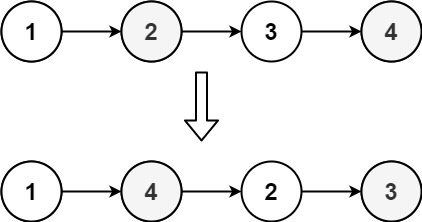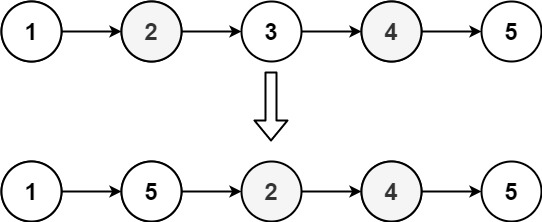Categories

## Hello world!

Welcome to WordPress. This is your first post. Edit or delete it, then start writing!

Categories

## 143. Reorder List Leetcode Javascript Solution

The Problem:

You are given the head of a singly linked-list. The list can be represented as:

```L0 → L1 → … → Ln - 1 → Ln
```

Reorder the list to be on the following form:

```L0 → Ln → L1 → Ln - 1 → L2 → Ln - 2 → …
```

You may not modify the values in the list’s nodes. Only nodes themselves may be changed.

Example 1:```Input: head = [1,2,3,4]
Output: [1,4,2,3]
```

Example 2:```Input: head = [1,2,3,4,5]
Output: [1,5,2,4,3]
```

Constraints:

• The number of nodes in the list is in the range `[1, 5 * 104]`.
• `1 <= Node.val <= 1000`

The Solution:

```var reorderList = function(head) {
while(fast.next && fast.next.next){
slow = slow.next
fast = fast.next.next
}
//console.log(fast.val)
p2 = slow.next
//console.log(p2)
slow.next = null
let prev = null
while(p2){
let next = p2.next
p2.next = prev
prev = p2
p2 = next
}
console.log(prev)
let part1 = head, part2 = prev
while(part2){
let p1 = part1.next
let p2 = part2.next
part1.next = part2
part2.next = p1
part1 = p1
part2 = p2
}
};
```
Categories

## 56. Merge Intervals Leetcode Javascript Solution

The Problem:

Given an array of `intervals` where `intervals[i] = [starti, endi]`, merge all overlapping intervals, and return an array of the non-overlapping intervals that cover all the intervals in the input.

Example 1:

```Input: intervals = [[1,3],[2,6],[8,10],[15,18]]
Output: [[1,6],[8,10],[15,18]]
Explanation: Since intervals [1,3] and [2,6] overlaps, merge them into [1,6].
```

Example 2:

```Input: intervals = [[1,4],[4,5]]
Output: [[1,5]]
Explanation: Intervals [1,4] and [4,5] are considered overlapping.
```

Constraints:

• `1 <= intervals.length <= 104`
• `intervals[i].length == 2`
• `0 <= starti <= endi <= 104`

The Problem:

```var merge = function(intervals) {
if(intervals.length == 1 || intervals.length == 0){ return intervals}
intervals.sort((a,b) => a !== b ? a-b : a-b)
// console.log(intervals)
let ans = [], prev = intervals
ans.push(prev)
for(let interval of intervals){
// console.log(prev)
if(interval <= prev){
prev = Math.max(interval, prev)
//prev = interval (remember not to update prev in this if
} else {
ans.push(interval)
prev = interval
}
// console.log(ans)
}
return ans
};```
Categories

## 1721. Swapping Nodes in a Linked List Leetcode Javascript Solution

The Problem:

You are given the `head` of a linked list, and an integer `k`.

Return the head of the linked list after swapping the values of the `kth` node from the beginning and the `kth` node from the end (the list is 1-indexed).

Example 1:

```Input: head = [1,2,3,4,5], k = 2
Output: [1,4,3,2,5]
```

Example 2:

```Input: head = [7,9,6,6,7,8,3,0,9,5], k = 5
Output: [7,9,6,6,8,7,3,0,9,5]
```

Example 3:

```Input: head = , k = 1
Output: 
```

Example 4:

```Input: head = [1,2], k = 1
Output: [2,1]
```

Example 5:

```Input: head = [1,2,3], k = 2
Output: [1,2,3]
```

Constraints:

• The number of nodes in the list is `n`.
• `1 <= k <= n <= 105`
• `0 <= Node.val <= 100`

The Solution:

I got stuck at trying a new element swapping method with ES6 and wasted a good amount of time. In the end, I printed out variables line by line and used the old swapping method.

```var swapNodes = function(head, k) {
let dummy = new ListNode(0)
let fast = dummy, slow = dummy
for(let i = 1; i <= k; i++){
fast = fast.next
}
//  console.log(fast.val)
let first = fast
while(fast.next != null){
slow = slow.next
fast = fast.next
}
let second = slow.next
//console.log("first: " + first.val + " second: " + second.val)
// first.val, second.val = second.val, first.val
//let a = 2, b = 4
let temp = first.val
first.val = second.val
second.val = temp
// console.log( "a: " + a + " b: " + b)
// console.log("first: " + first.val + " second: " + second.val)
return dummy.next
};```
Categories

## 438. Find All Anagrams in a String Leetcode Javascript Solution

The Problem:

Given a string s and a non-empty string p, find all the start indices of p‘s anagrams in s.

Strings consists of lowercase English letters only and the length of both strings s and p will not be larger than 20,100.

The order of output does not matter.

Example 1:

```Input:
s: "cbaebabacd" p: "abc"
Output:
[0, 6]
Explanation:
The substring with start index = 0 is "cba", which is an anagram of "abc".
The substring with start index = 6 is "bac", which is an anagram of "abc".
```

Example 2:

```Input:
s: "abab" p: "ab"
Output:
[0, 1, 2]
Explanation:
The substring with start index = 0 is "ab", which is an anagram of "ab".
The substring with start index = 1 is "ba", which is an anagram of "ab".
The substring with start index = 2 is "ab", which is an anagram of "ab".```

The Solution:

```var findAnagrams = function(s, p) {
let set = new Map()
for(let i = 0; i < p.length; i++){
if(!set.has(p[i])){
set.set(p[i],1)
} else{
set.set(p[i],set.get(p[i]) + 1)
}
}
// console.log(set)
let ans = []
let left = 0, right = 0, count = set.size
while (right < s.length){
if(set.has(s[right])){
set.set(s[right],set.get(s[right]) - 1)
if(set.get(s[right])== 0){
count--
}
}
while(count == 0){
if(set.has(s[left])){
set.set(s[left],set.get(s[left]) + 1)
if(set.get(s[left]) > 0){
count++
}
}
if(right - left +1 == p.length){
ans.push(left)
}
left++
}
right++
}
return ans
};```
Categories

## 236. Lowest Common Ancestor of a Binary Tree Leetcode Javascript Solution

The Problem:

Given a binary tree, find the lowest common ancestor (LCA) of two given nodes in the tree.

According to the definition of LCA on Wikipedia: “The lowest common ancestor is defined between two nodes p and q as the lowest node in T that has both p and q as descendants (where we allow a node to be a descendant of itself).”

Example 1:

```Input: root = [3,5,1,6,2,0,8,null,null,7,4], p = 5, q = 1
Output: 3
Explanation: The LCA of nodes 5 and 1 is 3.
```

Example 2:

```Input: root = [3,5,1,6,2,0,8,null,null,7,4], p = 5, q = 4
Output: 5
Explanation: The LCA of nodes 5 and 4 is 5, since a node can be a descendant of itself according to the LCA definition.
```

Example 3:

```Input: root = [1,2], p = 1, q = 2
Output: 1
```

Constraints:

• The number of nodes in the tree is in the range `[2, 105]`.
• `-109 <= Node.val <= 109`
• All `Node.val` are unique.
• `p != q`
• `p` and `q` will exist in the tree.

The Solution:

```var lowestCommonAncestor = function(root, p, q) {
if(!root || root== p || root == q) return root
let left = lowestCommonAncestor(root.left,p,q)
let right = lowestCommonAncestor(root.right,p,q)
return (left && right) ? root : (left || right)
};```
Categories

## 47. Permutations II Leetcode Javascript Solution

The Problem:

Given a collection of numbers, `nums`, that might contain duplicates, return all possible unique permutations in any order.

Example 1:

`Input: nums = [1,1,2]Output:[[1,1,2], [1,2,1], [2,1,1]]`

Example 2:

`Input: nums = [1,2,3]Output: [[1,2,3],[1,3,2],[2,1,3],[2,3,1],[3,1,2],[3,2,1]]`

Constraints:

• `1 <= nums.length <= 8`
• `-10 <= nums[i] <= 10`

The Solution:

For this kind of problem, we’ll need to use backtracking. We also implement a boolean array with the same length as that of the number array to keep track of visited elements (because the number array contains duplicates):

```var permuteUnique = function(nums) {
let results = []
nums.sort((a,b) => a-b)
function backtrack(nums,results, temp,used){
if(temp.length == nums.length){
results.push([…temp])
}
for(let i = 0; i < nums.length; i++){
// console.log(used + “ “+ i + “ “ + temp)
if(used[i] || (i > 0 && nums[i] == nums[i-1] && used[i — 1]) ){continue}
used[i] = true
temp.push(nums[i])
backtrack(nums,results, temp, used)
used[i] = false
temp.pop()
}
}
backtrack(nums,results, new Array(), new Array(nums.length).fill(false))
return results
};```
Categories

## 46. Permutations Leetcode Javascript Solution

The Problem:

Given an array `nums` of distinct integers, return all the possible permutations. You can return the answer in any order.

Example 1:

```Input: nums = [1,2,3]
Output: [[1,2,3],[1,3,2],[2,1,3],[2,3,1],[3,1,2],[3,2,1]]
```

Example 2:

```Input: nums = [0,1]
Output: [[0,1],[1,0]]
```

Example 3:

```Input: nums = 
Output: []
```

Constraints:

• `1 <= nums.length <= 6`
• `-10 <= nums[i] <= 10`
• All the integers of `nums` are unique.

The Solution:

We use backtracking:

```var permute = function(nums) {
let results = []
function backtrack(nums, results, temp){
if(temp.length == nums.length){
results.push([...temp])
}
for(let i = 0 ; i < nums.length; i++){
if(temp.includes(nums[i])){continue}
temp.push(nums[i])
backtrack(nums,results,temp)
temp.pop()
}
}
backtrack(nums,results, new Array())
return results
};```
Categories

## 90. Subsets II Leetcode Javascript Solution

The Problem:

Given an integer array `nums` that may contain duplicates, return all possible subsets (the power set).

The solution set must not contain duplicate subsets. Return the solution in any order.

Example 1:

```Input: nums = [1,2,2]
Output: [[],,[1,2],[1,2,2],,[2,2]]
```

Example 2:

```Input: nums = 
Output: [[],]
```

Constraints:

• `1 <= nums.length <= 10`
• `-10 <= nums[i] <= 10`

The Solution:

```var subsetsWithDup = function(nums) {
let results = [],temp = []
nums.sort((a,b) => a-b)
console.log(nums)
function backtrack(nums,start,results, temp){
results.push([...temp])
/* console.log("results " )
for( rest of results){
console.log(rest)
}
*/
for(let i = start; i < nums.length; i++){
//console.log("i " + i)
if(i == start || nums[i] != nums[i-1]){
//                 console.log("i after if" + i)
temp.push(nums[i])
//   console.log(temp)
backtrack(nums,i+1,results,temp)
temp.pop()
//                console.log("temp after pop " + temp)
}
}
}
backtrack(nums,0,results,temp)
return results
};```
Categories

## 78. Subsets Leetcode Javascript Solution

The Problem:

Given an integer array `nums` of unique elements, return all possible subsets (the power set).

The solution set must not contain duplicate subsets. Return the solution in any order.

Example 1:

```Input: nums = [1,2,3]
Output: [[],,,[1,2],,[1,3],[2,3],[1,2,3]]
```

Example 2:

```Input: nums = 
Output: [[],]
```

Constraints:

• `1 <= nums.length <= 10`
• `-10 <= nums[i] <= 10`
• All the numbers of `nums` are unique.

The Solution:

We use backtracking for this problem.

```var subsets = function(nums) {
let results = []
nums.sort()
backtrack(nums,0,results,new Array())
function backtrack(nums, start, results, temp){
results.push([...temp])
for(let i = start; i < nums.length; i++){
temp.push(nums[i])
backtrack(nums,i+1,results,temp)
temp.pop()
}
}
return results
};```